Definitions of Square Dance Calls and Concepts

Index -->  Plus  |  A1  |  A2  |  C1  |  C2  |  C3A  |  C3B  |  C4  |  NOL  |
Definitions (Text Only) -->  Plus  |  A1  |  A2  |  C1  |  C2  |  C3A  |  C3B  |  C4  |  NOL  |
 Find call:

Magic Diamond Concept -- [C4]
(Dave Hodson 1979)
 C4:Language: \$B8@8l(B Språk: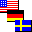All view (admin)

From Twin Diamonds. 2 \$B\$D\$N(B Diamond \$B\$+\$i(B. Från Twin Diamonds.

A Magic Diamond is a Distorted Diamond in which the Centers of one Diamond work with the points of the other Diamond. Magic Diamond \$B\$O(B, 1 \$B\$D\$N(B diamond \$B\$N(B Centers \$B\$,B>\$N(B Diamond \$B\$N(B points \$B\$HF0\$/(B Distorted (\$B\$f\$,\$s\$@(B) Diamond \$B\$G\$9(B. En Magic Diamond är en distorderad Diamond där Centers på en Diamond arbetar med points i den andra Diamond.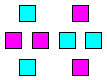Work with these dancers in a distorted Diamond. \$B\$3\$l\$i\$N%@%s%5!<\$,(B distorted (\$B\$f\$,\$s\$@(B) Diamond \$B\$GF0\$-\$^\$9(B. Arbeta med dessa dansare i en distorderad Diamond.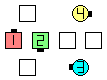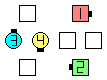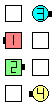before före Magic Diamond Circulate\$B\$NA0(B after efter \$B8e(B Now, from here,Magic Flip The Diamond \$B\$=\$7\$F(B, \$B\$3\$3\$+\$i(B,Magic Flip The Diamond \$B8e(B Nu, härifrån,Magic Flip The Diamond after efter \$B8e(B

Note that in the last diagram above you now have a Magic Wave [C4] with the dancers who were in your Magic Diamond. \$B\$3\$NNc\$G\$O(B, \$BCf\$N?^\$N(B Magic Diamond \$B\$K\$\$\$??M\$,(B, \$B1&\$N?^\$N(B Magic Wave [C4] \$B\$H\$J\$C\$F\$\$\$^\$9(B. Notera att i det sista diagrammet du nu har en Magic Wave [C4] med de två dansarna som var i din Magic Diamond.

Notes on traffic patterns: \$BDL\$jJ}\$K\$D\$\$\$F\$NCm Kommentarer till trafikmönstren:

• The basic rule is try to get to your ending position with a minimum number of collisions (trying for zero collisions would be a good goal). \$B4pK\E*\$J%k!<%k\$O(B, \$BB>\$N?M\$HF1\$80LCV\$K\$J\$k\$3\$H\$N2s?t\$,:G>.\$K\$J\$k\$h\$&\$K(B, \$B=*\$o\$j\$N0LCV\$X9T\$/\$3\$H\$G\$9(B (1 \$B2s\$bF1\$80LCV\$K\$J\$i\$J\$\$\$3\$H\$,NI\$\$\$G\$9(B). Grundregeln är att komma till din slutposition med ett minimum av kollisioner (att försöka med noll kollisioner vore en bra målsättning).
• On Magic Cut The Diamond or Magic Flip The Diamond, the points can often work as if In Tandem. Magic Cut The Diamond \$B\$^\$?\$O(B Magic Flip The Diamond \$B\$G\$O(B, points \$B\$OB?\$/\$N>l9g(B Tandem \$B\$GF0\$/\$3\$H\$,\$G\$-\$^\$9(B. Magic Cut The Diamond eller Magic Flip The Diamond, kan points ofta arbeta i Tandem.

In our opinion, callers should say Magic Diamond Cut | Flip The Diamond rather than Cut | Flip The Magic Diamond. In the first case, the dancers initially hear the word Magic and can immediately start locating their Magic Diamond. Also, in our opinion, Cut|Flip The Magic Diamond is NOT the same as Magic Cut|Flip The Diamond, since on a Cut|Flip The formation those with handholds do a formation Circulate as the others simply slide together and Trade (Cut) or Phantom Run (Flip). Hence, for a Cut|Flip The Magic Diamond only the Centers are working Magic. \$B;dC#\$N0U8+\$G\$O(B, \$B%3!<%i!<\$O(B Cut | Flip The Magic Diamond \$B\$G\$J\$/(B Magic Diamond Cut | Flip The Diamond \$B\$H8@\$&\$Y\$-\$G\$9(B. \$B\$=\$&\$9\$l\$P(B, \$B%@%s%5!<\$O;O\$a\$K(B Magic \$B\$HJ9\$/\$N\$G(B, \$B<+J,\$N(B Magic Diamond \$B\$r\$9\$0A\\$7;O\$a\$k\$3\$H\$,\$G\$-\$^\$9(B. \$B\$^\$?(B, \$B;dC#\$N0U8+\$G\$O(B, Cut|Flip The Magic Diamond \$B\$O(B Magic Cut|Flip The Diamond \$B\$HF1\$8\$G\$O(B\$B\$"\$j\$^\$;\$s(B. \$B\$J\$<\$J\$i(B, Cut|Flip The formation \$B\$G\$O(B, \$Bformation Circulate \$B\$r\$7(B, \$BB>\$N?M\$OC1\$K\$*8_\$\$\$K6a\$E\$\$\$F(B Trade (Cut \$B\$N\$H\$-(B) \$B\$^\$?\$O(B Phantom Run (Flip \$B\$N\$H\$-(B) \$B\$r\$7\$^\$9(B. \$B\$7\$?\$,\$C\$F(B, Cut|Flip The Magic Diamond \$B\$G\$O(B Centers \$B\$@\$1\$,(B Magic \$B\$GF0\$/\$3\$H\$K\$J\$j\$^\$9(B. Vi anser att caller bör säga Magic Diamond Cut | Flip The Diamond istället för Cut | Flip The Magic Diamond. I det första fallet där dansarna först hör ordet Magic kan de omedelbart börja lokalisera sin Magic Diamond. Dessutom anser vi, att Cut|Flip The Magic Diamond INTE är samma som Magic Cut|Flip The Diamond, eftersom på en Cut|Flip The formation gör de som har handfattning en formation Circulate medan de andra helt enkelt glider samman och Trade (Cut) eller Phantom Run (Flip). Således, på en Cut|Flip The Magic Diamond skulle bara Centers arbeta Magic.

Magic Inpoint|Outpoint|Inside|Outside Triangle Identification: Identifiering: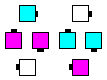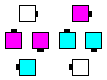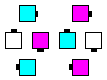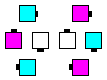MagicInpoint Triangle MagicOutpoint Triangle MagicInside Triangle MagicOutside Triangle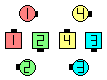Dancers are listed in order of their Circulate path within the above diagram: Dansarna är listade i ordning i sin Circulate bana i ovanstående diagram:

• Magic Inpoint Triangle: (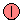,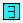,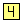) and \$B\$H(B och (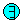,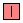,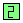)
• Magic Outpoint Triangle: (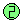,,) and \$B\$H(B och (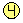,,)
• Magic Inside Triangle: (,,) and \$B\$H(B och (,,)
[This is the hardest one to see.] [\$B\$3\$l\$O8+6K\$a\$k\$3\$H\$,0lHVFq\$7\$\$\$b\$N\$G\$9(B.] [Den här är svårast att se.]
• Magic Outside Triangle: (,,) and \$B\$H(B och (,,)

Magic Interlocked Diamond Concept [C4]: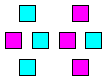Work with these dancers in a distorted Diamond. \$B\$3\$l\$i\$N%@%s%5!<\$,(B distorted (\$B\$f\$,\$s\$@(B) Diamond \$B\$GF0\$-\$^\$9(B. Arbeta med dessa dansare i en distorderad Diamond.

Magic Interlocked Diamonds are rarely used since they are difficult to visualize and often involve hideous traffic patterns. Magic Interlocked Diamonds \$B\$O\$[\$H\$s\$I;H\$o\$l\$^\$;\$s(B. \$B\$J\$<\$J\$i\$P(B, \$B8+\$D\$1\$k\$3\$H\$,Fq\$7\$\$\$3\$H\$H(B, \$B\$7\$P\$7\$P\$R\$I\$\$DL\$jJ}\$K\$J\$k\$+\$i\$G\$9(B. Magic Interlocked Diamonds används sällan eftersom trafikmönstret blir gräsligt.Comments? Questions? Suggestions?
 C4: